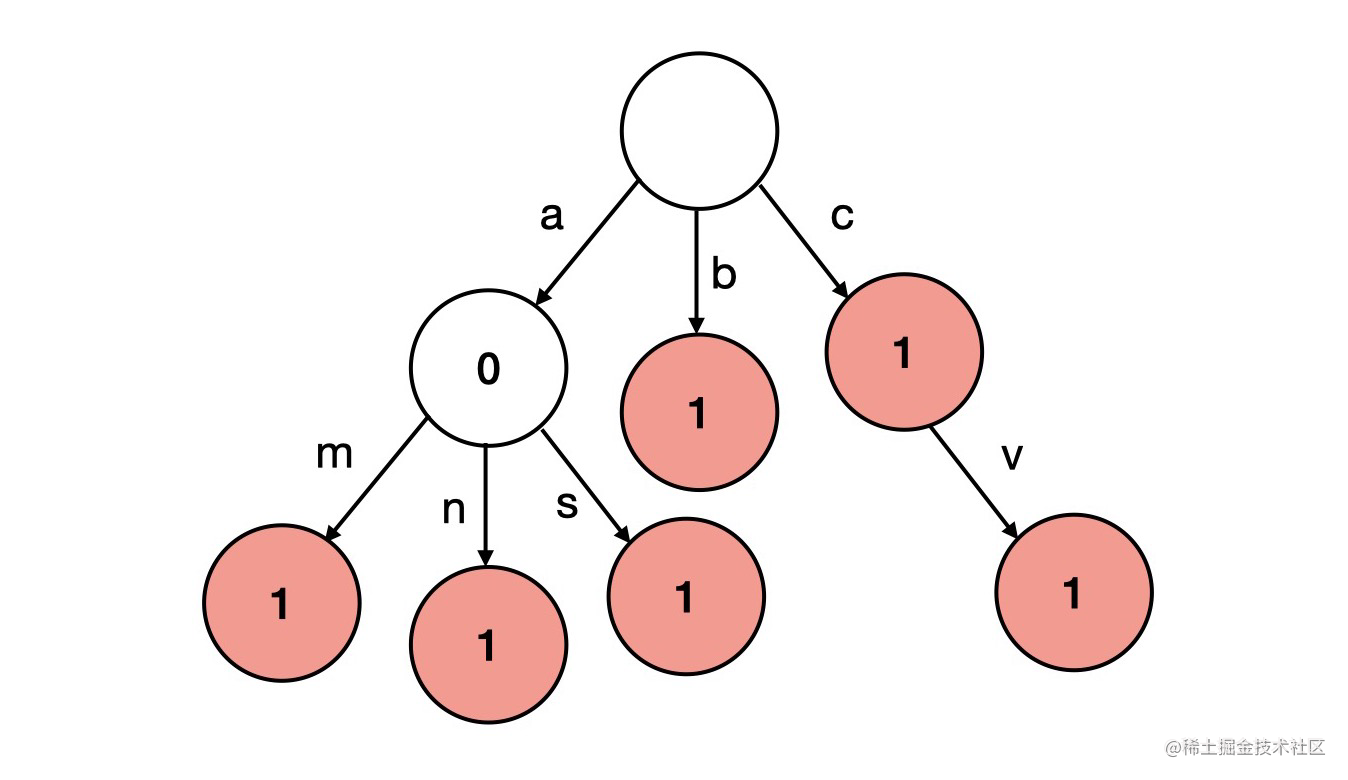# 1、最大子序和

## 思路

• 动态规划的是首先对数组进行遍历，当前最大连续子序列和为 sum，结果为 ans

• 如果 sum > 0，则说明 sum 对结果有增益效果，则 sum 保留并加上当前遍历数字

• 如果 sum <= 0，则说明 sum 对结果无增益效果，需要舍弃，则 sum 直接更新为当前遍历数字

• 每次比较 sum 和 ans的大小，将最大值置为ans，遍历结束返回结果

• 时间复杂度：O(n)O(n)

## 题解

``````var maxSubArray = function(nums) {
let ans = nums;
let sum = 0;
for(const num of nums) {
if(sum > 0) {
sum += num;
} else {
sum = num;
}
ans = Math.max(ans, sum);
}
return ans;
};

# 2、Excel表列名称

## 题解

``````var convertToTitle = function(columnNumber) {
let sb = '';
while(columnNumber > 0){
columnNumber --;
sb = sb + String.fromCharCode(columnNumber%26 + 'A'.charCodeAt(0));
columnNumber = Math.floor(columnNumber/26);
}
sb = sb.split('').reverse().join('');
return sb;
};

# 3、实现Trie（前缀树）

## 题目

Trie
（发音类似 "try"）或者说 前缀树 是一种树形数据结构，用于高效地存储和检索字符串数据集中的键。这一数据结构有相当多的应用情景，例如自动补完和拼写检查。

• `Trie()` 初始化前缀树对象。
• `void insert(String word)` 向前缀树中插入字符串 `word` 。
• `boolean search(String word)` 如果字符串 `word` 在前缀树中，返回 `true`（即，在检索之前已经插入）；否则，返回 `false` 。
• `boolean startsWith(String prefix)` 如果之前已经插入的字符串 `word` 的前缀之一为 `prefix` ，返回 `true` ；否则，返回 `false` 。

## 思路

### 前缀树

（只保存小写字符的）「前缀树」是一种特殊的多叉树，它的 TrieNode 中 chidren 是一个大小为 26 的一维数组，分别对应了26个英文字符 'a' ~ 'z'，也就是说形成了一棵 26叉树。

value 表示当前的单词就是当前的value值。 children 是该节点的所有子节点。 end 表示当前节点是否是单词的结束。

``````class Node {
constructor(value){
this.value = value;
this.children = {};
this.end = false;
}
}

### 构建## 题解

``````class Trie {
constructor(){
this.root = new Node()
}
insert(word){
let currentNode = this.root;
for (const c of word) {
if (!currentNode.children[c]) {
currentNode.children[c] = new Node(c);
}
currentNode = currentNode.children[c];
}
currentNode.end = true;
}
startsWith(word){
let currentNode = this.root;
for(const c of word) {
if(!currentNode.children[c]){
return false;
}
currentNode = currentNode.children[c];
}
return true;

}
search(word){
let currentNode = this.root;
for(const c of word) {
if(!currentNode.children[c]){
return false;
}
currentNode = currentNode.children[c];
}
return currentNode.end;
}
}

# 4、删除排序列表中的重复元素

## 思路

• 标签：链表
• 当 cur 和 cur.next 的存在为循环结束条件，当二者有一个不存在时说明链表没有去重复的必要了
• 当 cur.val 和 cur.next.val 相等时说明需要去重，则将 cur 的下一个指针指向下一个的下一个，这样就能* 达到去重复的效果
• 如果不相等则 cur 移动到下一个位置继续循环
• 时间复杂度：O(n)O(n)

## 题解

``````/**
* function ListNode(val, next) {
*     this.val = (val===undefined ? 0 : val)
*     this.next = (next===undefined ? null : next)
* }
*/
/**
* @return {ListNode}
*/
while(cur!= null && cur.next != null){
if(cur.val === cur.next.val){
cur.next = cur.next.next;
}else{
cur = cur.next
}
}
};

# 5、回文对

## 题解

``````/**
* @param {string[]} words
* @return {number[][]}
*/
var palindromePairs = function(words) {
let result = [];
for(let i = 0;i<words.length;i++){
for(let j = 0;j<words.length;j++){
if(i===j){
continue
}
if(is_palindromePairs(words[i]+words[j])){
result.push([i,j])
}
}
}
return result;
};

const is_palindromePairs = function(s){
for(let i = 0;i<s.length/2;i++){
if(s[i]!= s[s.length-1-i]){
return false
}
}
return true;
}